# Curvahedra Geometry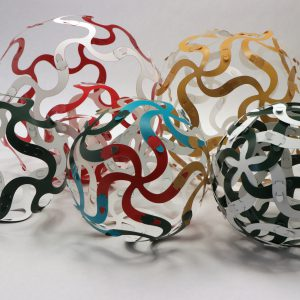Longtime friend of the Aperiodical, artist, mathematician and #BigMathOff semifinalist Edmund Harriss has come up with a new puzzle/toy/exploration set, developing his Curvahedra system. We asked him to explain the maths behind it in this guest post.

Curvahedra is a flexible system of connectors that can make all sorts of different things, combining puzzles (and self-created puzzles) with art. You can get your own to play with, explore, prepare for Christmas (they make great decorations, wreaths and presents) at our online store, and get 15% off with the discount code APERIODICAL.

As this is the Aperiodical, you might be most interested in how it can be used to explore mathematics. In the big math off I talked about the basic ideas behind the system, Gauss’ famous Theorema Egregium and Gauss-Bonnet theorems. A really simple version of this comes from just considering triangles, that can be built up to make this:

To begin this story we need to think about an equilateral triangle: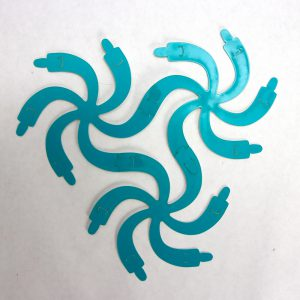This is a bit of a cheat for a triangle as the edges are curved, but the edges do form an ‘S’ starting and stopping in the same direction, so as we go round the triangle the only place we turn is the three corners. Each of these has six symmetric arms. So the angles will be 60 degrees. Three such angles of course gives $180$. Extending this triangle out gives a pretty, flat plane pattern: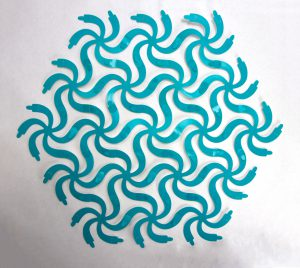What happens if we take an arm away? Just five arms at each corner, the triangle now has $3 \times 72$ degrees, which is $216$ – a little too much: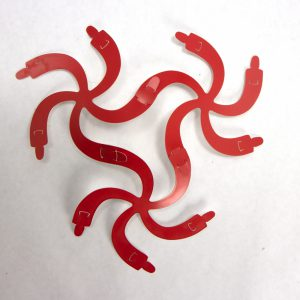As you can see this bad triangle now does not lie flat but bends up. Now when we keep connecting triangles the result continues to curve round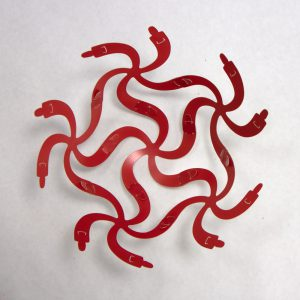Building up into a sphere: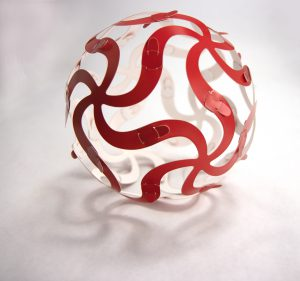This will be familiar to many as a spherical icosahedron. Unlike the polyhedral version, with flat faces and sharp edges and corners, this one bends smoothly into a sphere. The curvature of the surface happening in the gaps between pieces. Now we can look at that extra angle, each triangle had an excess of $36$ degrees. There are 20 triangles so the total spare angle is $720$ degrees. Keep that number in mind.

Let’s take another branch away: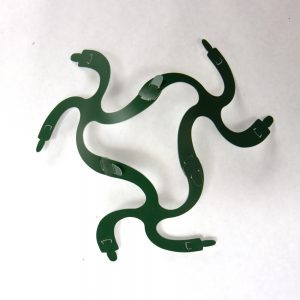With four arms the angle is 90 degrees. So the triangle now has $3 \times 90= 270$ degrees, an excess of $90$. Building out with these triangles we again get a ball: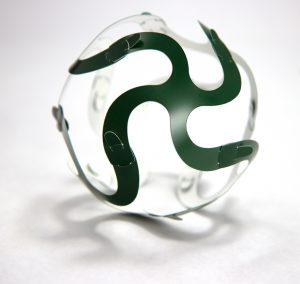This new ball has eight triangles, so the total excess is $8 \times 90$, again $720$. This is not a coincidence, and is related to one of the earliest results from what would become the study of topology, Descartes’ theorem. If we started with 3-branch pieces and again made triangles can you work out how many triangles we would end up with?

You can play the same game with a torus like this one, named in a bad pun Taurus, one of the kits you can buy. (We did say that the system would make a good wreath!):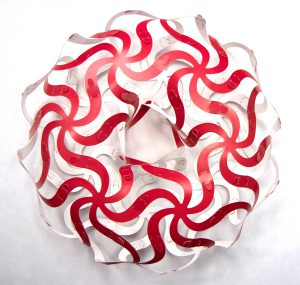This gets a little more complicated as it has triangles and quadrilaterals, however the final sum adds up not to $720$, but to $0$. That is because some of the shapes have too little angle, not too much and everything balances out. What happens when every shape has too little angle? A simple way to do this is to extend the sequence $4,5,6$ by $1$, starting with 7-branch pieces: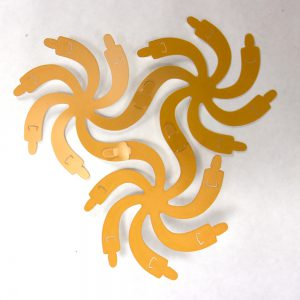The result again curves, but not in a single direction like the previous examples, it waves up and down, not unlike a saddle. Continuing with more triangles the surface gets more wobbly: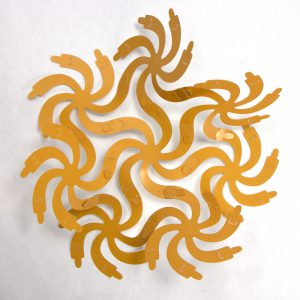This is not just a saddle, but a surface with a saddle at every point, so as you build out the amount of material added gets greater and greater, creating the surface we started with: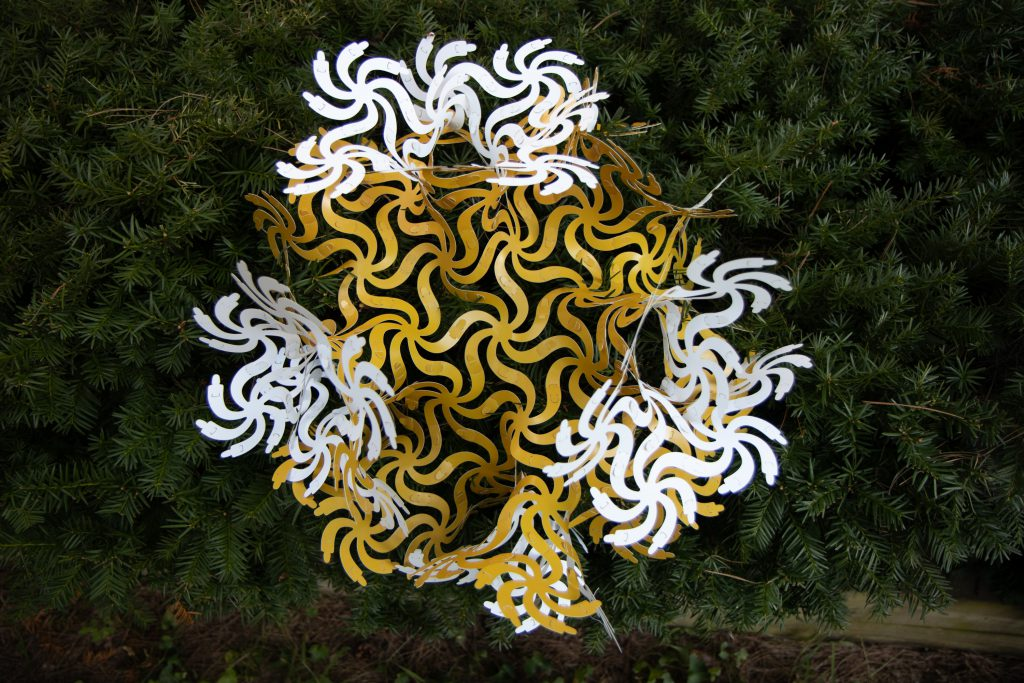This object is a model for the mysterious mathematics of hyperbolic geometry, as well as giving hints for how nature makes surfaces with a high surface area to volume ratio like Corals or our brains. Yet we can start to think about it just by connecting some triangle pieces together so that everything lies flat locally.

What will you explore with Curvahedra?

•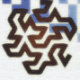#### Edmund Harriss

Mathematician, Teacher, Artist and Maker at the Uni of Arkansas. Author of Patterns/Visions of the Universe with Alex Bellos, and creator of Curvahedra.

### One Response to “Curvahedra Geometry”

1.cricktutors

Wow! Very interesting to hear this. I wanna get one. Is there any available site to buy this?